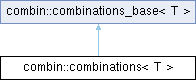Cadabra Computer algebra system for field theory problems
combin::combinations< T > Class Template Reference

#include <Combinatorics.hh>

Inheritance diagram for combin::combinations< T >:## Public Types

typedef std::vector< std::vector< T > > permuted_sets_t

typedef permuted_sets_t::const_iterator const_iteratorPublic Types inherited from combin::combinations_base< T >
enum  weight_cond { weight_equals, weight_less, weight_greater }

## Public Member Functions

combinations ()

combinations (const std::vector< T > &)

virtual ~combinations ()

virtual void clear ()

virtual void clear_results ()

const std::vector< T > & operator[] (unsigned int) const

int ordersign (unsigned int) const

unsigned int size () const

unsigned int multiplier (unsigned int) constPublic Member Functions inherited from combin::combinations_base< T >
combinations_base ()

combinations_base (const std::vector< T > &)

virtual ~combinations_base ()

void permute (long start=-1, long end=-1)

unsigned int sum_of_sublengths () const

void set_unit_sublengths ()

unsigned int multiplier (const std::vector< T > &) const

unsigned int total_permutations () const

## Protected Member Functions

virtual void vector_generated (const std::vector< unsigned int > &)Protected Member Functions inherited from combin::combinations_base< T >
virtual bool entry_accepted (unsigned int current) const

## Private Attributes

permuted_sets_t storagePublic Attributes inherited from combin::combinations_base< T >
unsigned int block_length

std::vector< unsigned int > sublengths

range_vector_t input_asym

std::vector< T > original

bool multiple_pick

std::vector< weights_tweights

std::vector< int > max_weights

std::vector< weight_condweight_conditions

unsigned int sub_problem_blocksizeProtected Attributes inherited from combin::combinations_base< T >
std::vector< unsigned int > temparr

long start_

long end_

long vector_generated_called_

std::vector< int > current_weight

## ◆ const_iterator

template<class T >
 typedef permuted_sets_t::const_iterator combin::combinations< T >::const_iterator

## ◆ permuted_sets_t

template<class T >
 typedef std::vector > combin::combinations< T >::permuted_sets_t

## ◆ combinations() [1/2]

template<class T >

## ◆ combinations() [2/2]

template<class T >
 combin::combinations< T >::combinations ( const std::vector< T > & oa )

## ◆ ~combinations()

template<class T >
 combin::combinations< T >::~combinations
virtual

## ◆ clear()

template<class T >
 void combin::combinations< T >::clear
virtual

Reimplemented from combin::combinations_base< T >.

## ◆ clear_results()

template<class T >
 void combin::combinations< T >::clear_results
virtual

Reimplemented from combin::combinations_base< T >.

## ◆ multiplier()

template<class T >
 unsigned int combin::combinations< T >::multiplier ( unsigned int num ) const

## ◆ operator[]()

template<class T >
 const std::vector< T > & combin::combinations< T >::operator[] ( unsigned int i ) const

## ◆ ordersign()

template<class T >
 int combin::combinations< T >::ordersign ( unsigned int num ) const

## ◆ size()

template<class T >
 unsigned int combin::combinations< T >::size

## ◆ vector_generated()

template<class T >
 void combin::combinations< T >::vector_generated ( const std::vector< unsigned int > & toadd )
protectedvirtual

## ◆ storage

template<class T >
 permuted_sets_t combin::combinations< T >::storage
private

The documentation for this class was generated from the following file: